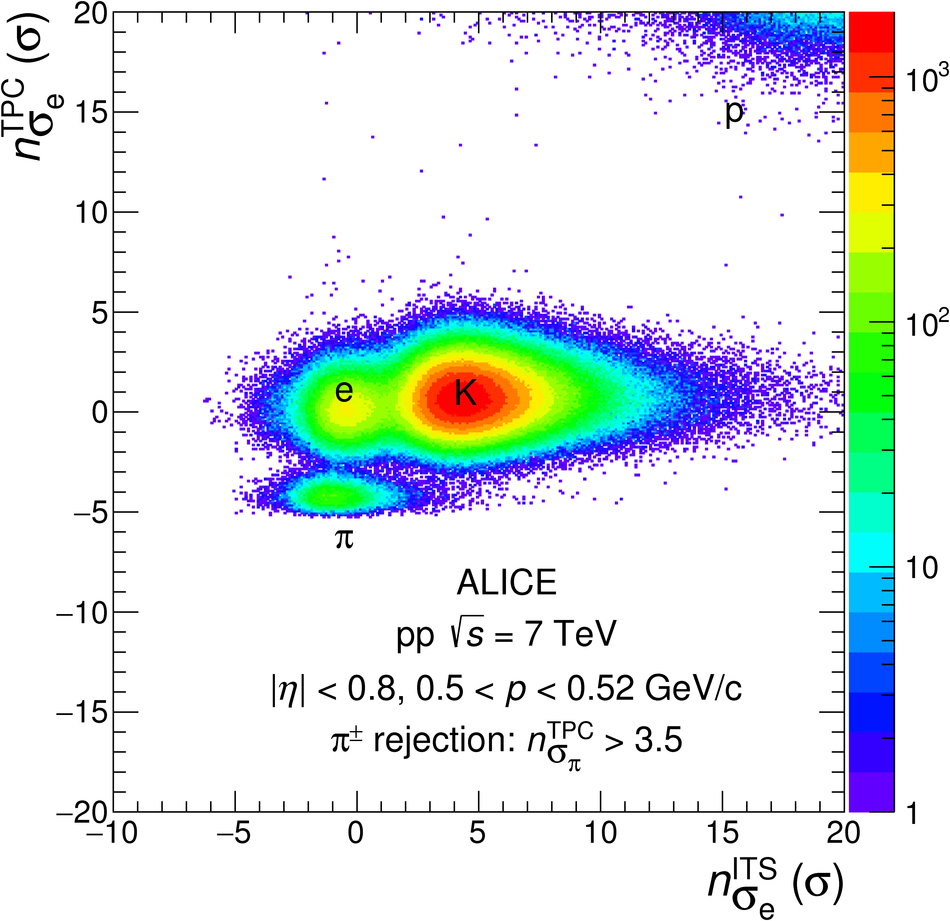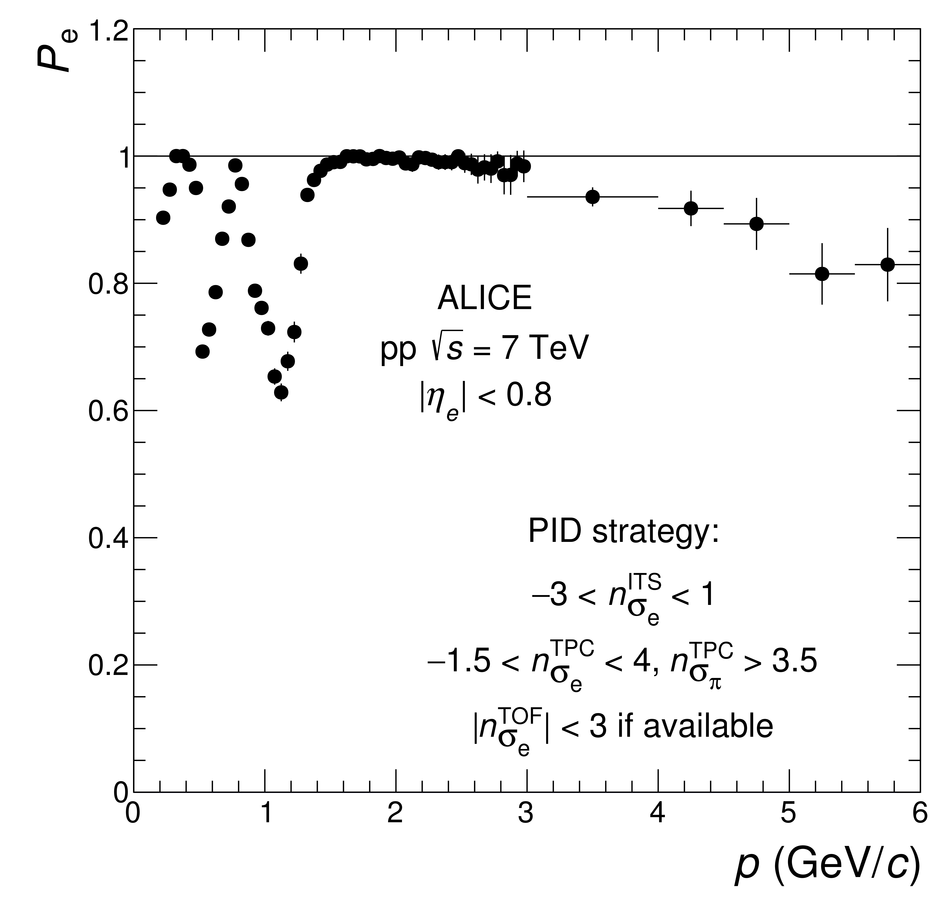# Figure 1

 TPC PID signal expressed as \sTPCe (see text) as a function of the ITS PID signal ($n^{\rm ITS}_{\sigma_{{\rm{e}}}}$) for selected tracks with 0.5 $<$ $p$ $<$ 0.52~\GeVoverc after applying the pion rejection in the TPC (left). Electron purity $P_{\rm e}$ as a function of momentum (right). Only statistical uncertainties are shown.